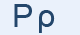Greek Letter Rho (Ρο - Ρ)The Greek letter came from the Semitic form, and for some reason the Romans added a diagonal bar to distinguish this from their altered form of "pi", which they used for the "p" sound.

"Ρ" Letter in mathematics and in science

ρ represents:

• the radius in a polar coordinate system
• the correlation coefficient in statistics
• the sensitivity to interest rate in mathematical finance
• density (mass per unit volume)
• resistivity
• the shape and reshape operators in APL

Greek words with "ρ"

ρόδα, πόρτα